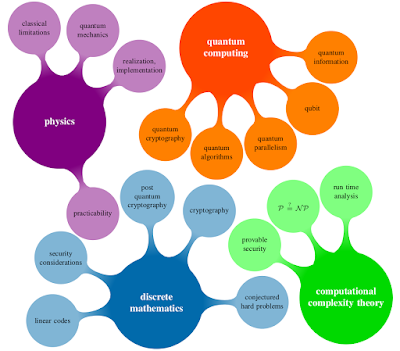## Friday, December 18, 2015

### Codes and Coding

as my master's thesis was about Linear Codes and Applications in Cryptography I'd like to provide some content of it in a nutshell and to give some recommendations of software that I used in the course of writing it.multiple scientific fields are involved (created using TikZ)
Modern cryptography covers among others the three areas privacy, authentication and integrity of messages. The aim of coding theory on the other hand described by one word is reliability - adding redundancy to messages in order to detect transmission errors. Both fields cover seemingly different areas in the field of digital information processing.

The motivation of my thesis was to showcase the combination of multiple scientific fields within coding theory and modern cryptography and to explore how they interact in the domain mentioned above and why they are a good choice.

In the center of attention stood the McEliece public-key cryptosystem, it's variation (Niederreiter's cryptosystem) and generalizations to other code classes after those code classes of interest were introduced and discussed.

The McEliece public-key cryptosystem was published in 1978, is based on disguising a special code as a general code and it is of interest back then and today because it seems to stand solid in the so-called post-quantum era, when a powerful quantum computer is available to attackers. More than 35 years ago the original code parameters: codeword length $n = 1024$, code dimension (or message bits) $k = 524$ and error-correcting capability $t = 50$ were proposed for the binary code underlying the McEliece public-key cryptosystem for $2^64$ bit security. To achieve the a reasonable security level today they have been adjusted to $n = 6960, k = 5413, t = 119$ because of attacks that were explored since then.

Given the number $t$ of erroneous positions and a general code $C$, the task of decoding $y = c + e$ is to find a codeword $c \in C$ of Hamming weight or number of non-zero entries: $w(y-c) = w(e) = t$. In the complexity theoretic literature the result is that this problem is NP-complete - there is no algorithm known that fulfills this task in polynomial time depending on the input size (length of $y$ respectively $c$).

The choice to use a Goppa codes as private key, which is multiplied by permutation matrices to obtain the public key looking as if it was a general code, was due to performance reasons when decoding messages thanks to Patterson's algorithm.

Interestingly many attacks are not applicable to the class of binary Goppa codes that McEliece's scheme was based on but on similar schemes and generalizations allowing other alphabets or other structures - binary Goppa codes were seemingly proposed with foresight.

In spite of some advantages and the - so far - quantum computer resistant attacks the biggest drawback of public-key cryptosystems based on codes are rather long public keys compared to other schemes. If one adds a security margin, under the assumption of a working quantum computer performing the general attack through the application of Grover's algorithm, the public key size is as big as 1 MB.

In the thesis I implemented the fast algorithm (by N. J. Patterson) for algebraically decoding binary Goppa codes in Sage and demonstrated the full functionality of the system in yet another chapter giving an example. Since I was writing it all up in $\LaTeX$, using sagetex even allowed the computation and insertion of the results into the $\verb!.tex!$ file one the fly when the document is being compiled!

My recommendation (to my fellows) for researching, analyzing, developing & testing of algorithms in a structured manner with the ability to create nice plots and documents rather easily is to give Sage or Spyder a try. Even though it might not be a necessity in your research to actually code something, playing around with these tools already available is instructive and worth a try.

Sage is available online or offline via installation and it is a good choice if you need access to i.e. number theory. Maybe the aforementioned Spyder is preferable if a somewhat more automated handling even closer to the programming language Python of the implemented primitive at hand is needed in your case.Autumnal Bochum around Oct 31
As usual, a little plurivalent:
Happy Coding and Happy Halloween - because $Dec(25) = Oct(31)$, right?!